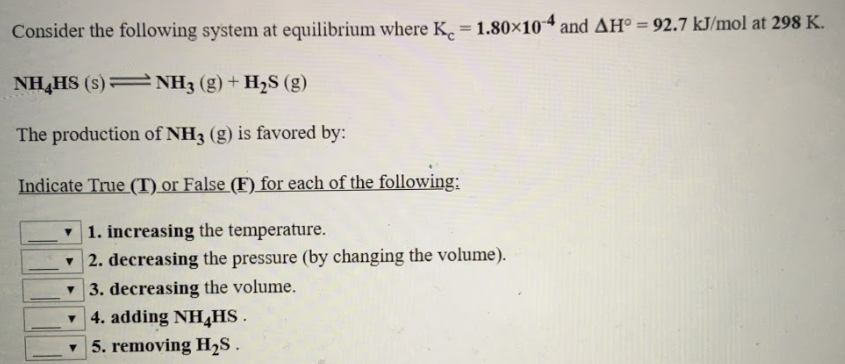# Consider the following system at equilibrium where Kc = 1.80x10^-4 and ΔH° = 92.7 kJ/mol at 298 K. NH4HS (s) ⇌ NH3 (g) + H2S (g) The production of NH3 (g) is favored by: Indicate True (T) of False (F) for each of the following: 1. increasing the temperature 2. decreasing the pressure (by changing the volume). 3. decreasing the volume 4. adding NH4HS 5. removing H2S